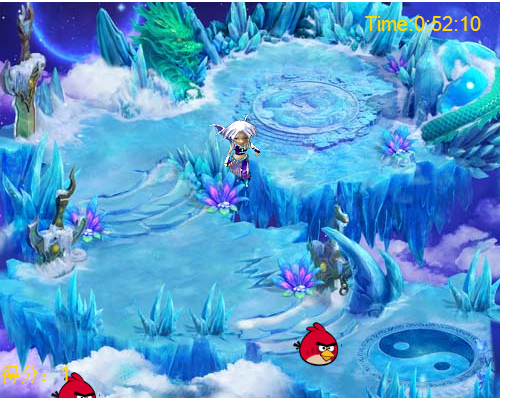# html5简单游戏编程从零开始

本文基于lufylegend游戏引擎，所以以下内容全部和此有关，

lufylegend引擎的下载地址： http://www.lufylegend.com/lufylegend

既然是使用引擎，首先就要配置引擎的开发环境，lufylegend的使用极其方便，只需将lufylegend-x.x.x.min.js文件引入即可，默认将legend文件夹放入当前文件路径中,如下方所示，

<!DOCTYPE html>
<html lang="en">
<meta charset="utf-8" />
<title>LTileMap</title>
<script type="text/javascript" src="lufylegend.js-lufylegend-1.9.7/lufylegend-1.9.7.min.js"></script>

此时即可开始使用lufylegend开始我们的游戏之旅

一、初始化引擎

加载引擎后，就需要对环境初始化，lufylegend的初始化需要使用引擎中提供的函数init(30,"legend",500,400,main);

第一个参数为刷新频率，也就是多久对屏幕刷新一次

第二个参数为需要初始化的html中的div的id，这也是lufylegend的优点之一

第三个参数为窗口的长

第四个参数为窗口的宽

第五个参数为初始化时调用的函数

二、游戏的图层

var backLayer,maplayer ,timelayer,playerlayer,moneylayer,pointlayer,gameoverLayer;

function gameinit(result){
//初始化分数
point=0;
imglist=result
//加入backlayer背景层
backLayer = new LSprite();
//加入地图层
maplayer= new LSprite();
//加入timelayer时间层
timelayer= new LTime(1);
timelayer.x=340;
timelayer.y=0;

//加入人物层
playerlayer= new LSprite();
//加入金币层
moneylayer= new LSprite();
//加入分数层
pointLayer= new LSprite();
//加入游戏结束层
gameoverLayer = new LSprite();
//初始化游戏
gamestart();
}

function LTime(min){
var s=this;
base(s,LSprite,[]);
s.x=1;
s.y=1;
s.time=min;
leftTime=s.time*60*1000;
time=new LTextField();
time.x=25;
time.y=10
time.size=15;
time.color="#ffD700";
s.onshow();
//s.onframe();
}

LTime为此类的构造函数，可以很清楚地看出此类继承自LSprite类，因此也具有精灵类的所有特性，在此基础上添加了一个用以显示倒计时的文字区域，x，y为文字起始位置的坐标，需要注意的是这里的x，y并不是针对整个游戏的坐标系来说的，而是针对所在类来看，如下图：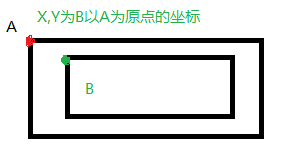LTime.prototype.onframe=function(){
//var s=this;
minutes=Math.floor(leftTime/1000/60);
second=Math.floor(leftTime/1000-60*minutes);
millsecond=Math.floor(leftTime-60*minutes*1000-1000*second);
mill=Math.floor(millsecond/10);
if(second<10){
second="0"+second;
}
if(mill<10){

mill="0"+mill;
}
time.text="Time:"+minutes+":"+second+":"+mill;
if(leftTime>0){
leftTime -= 1000*60/(1000*60/30);
}else{
return false;
}

}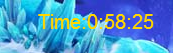//开始绘制游戏界面
function gamestart(result){
//加入地图
var bitmapData=new LBitmapData(imglist['map']);
var bitmap=new LBitmap(bitmapData);
//加入人物图片
anima=new LCharcter(imglist['cr']);
//加入记分牌
pointtext=new LTextField();
pointtext.x=0;
pointtext.y=360;
pointtext.size=15;
pointtext.color="#ffD700";
//加入动画和按键事件监听
}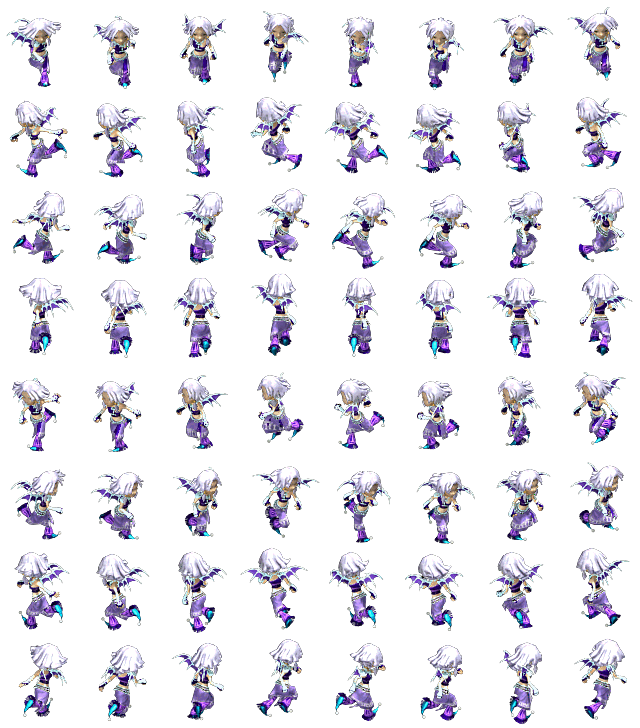function LCharcter(imgdata){
var s=this;
base(s,LSprite,[]);
s.x=200;
s.y=100;
s.imgdata=imgdata;
var list=LGlobal.divideCoordinate(640,728,8,8);
bitmapData=new LBitmapData(s.imgdata);
s.width=bitmapData.width/8;
s.height=bitmapData.height/8;
player= new LAnimation(s,bitmapData,list);
player.setAction(0,1,1,false);
}

LCharcter.prototype.move=function(moveto){
//仅设置一次action避免重复设置导致图形不断重置
var s=this;
if(moveto==40){
if(down==0){
player.setAction(0,1,1,false);
down++;
}
if(s.y<400-bitmapData.height){
s.y=s.y+speed;
}
}else if(moveto==39){
if(left==0){
player.setAction(2,1,1,false);
left++;
}
if(s.x<500-bitmapData.width){
s.x=s.x+speed;
}
}else if(moveto==38){
if(up==0){
player.setAction(3,1,1,false);
up++;
}
if(s.y>0){
s.y=s.y-speed;
}
}else if(moveto==37){
if(right==0){
player.setAction(1,1,1,false);
right++;
}
if(s.x>0){
s.x=s.x-speed;
}
}
}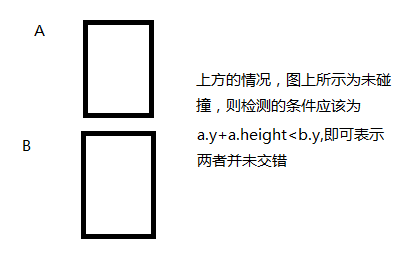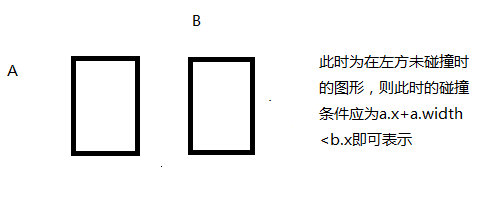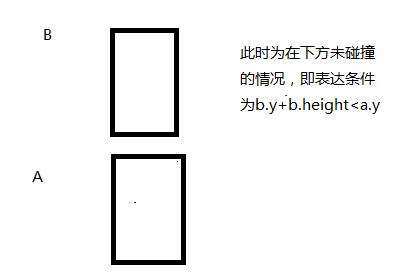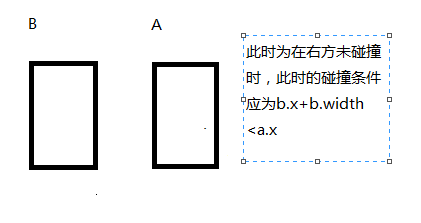LCharcter.prototype.CheckContact=function(money){
var s=this;
//money在右侧
if((s.x>money.x)&&(money.x+money.width<s.x)){
return false;
//money在左侧
}else if((s.x<money.x)&&(s.x+s.width<money.x)){

return false;
//money在下测
}else if((s.y<money.y)&&(s.y+s.height<money.y)){

return false;
//money在上测
}else if((s.y>money.y)&&(money.y+money.height<s.y)){
return false;
}else{
money.mode="die";
return true;
}
}

function onframe(){

//刷新分数
pointtext.text="得分："+point;
if(startflag==true){
var result=timelayer.onframe();

//刷新金币
if(result==false){
playerlayer.die();
//backLayer.die();
gameover();
}
for(i=0;i<moneylayer.childList.length;i++){
//让金币动起来
moneylayer.childList[i].run();
if(anima.CheckContact(moneylayer.childList[i])==true){
point++;
}
if(moneylayer.childList[i].mode == "die"){ //当金币移出屏幕或是与人物碰撞时……
//移除该成员
moneylayer.removeChild(moneylayer.childList[i]);
totalmoney++;
}
}

}
}